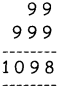Students can download 4th Maths Term 1 Chapter 2 Numbers Ex 2.1 Questions and Answers, Notes, Samacheer Kalvi 4th Maths Guide Pdf helps you to revise the complete Tamilnadu State Board New Syllabus, helps students complete homework assignments and to score high marks in board exams.

## Tamilnadu Samacheer Kalvi 4th Maths Solutions Term 1 Chapter 2 Numbers Ex 2.1

A. Write the following number in words:
(i) 1006 = ____________
(ii) 6327 = ____________
(iii) 9097 = ____________
(iv) 10,000 = ____________
(v) 8906 = ____________
(i) 1006 = One thousand and six
(ii) 6327 = six thousand three hundred and twenty seven
(iii) 9097 = nine thousand and ninety seven
(iv) 10,000 = ten thousand
(V) 8906 = eight thousand nine hundred and sixB. Write the numeral for each of the following.
(i) Seven thousand and sixty four = ________
(ii) Nine thousand three hundred and forty = ________
(iii) Five thousand six hundred and seventy three = ________
(iv) Ten thousand = ________
(v) Four thousand three hundred and six = ________
(i) Seven thousand and sixty four = 7064
(ii) Nine thousand three hundred and forty = 9340
(iii) Five thousand six hundred and seventy three = 5673
(iv) Ten thousand = 10,000
(v) Four thousand three hundred and six = 4306